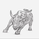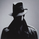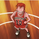23377 vues
As we all know, as published by Wilder, RSI makes use of "CLOSE" values. You probably have experimented changing the input to hl2 or hlc3 . I have included many other RSI variations in this chart. Refer to the developers section below to learn how you can use this code in your scripts.

1) RSI with Volume
---------------------------------------------
Suggested by Morris, this idea adds volume to the RSI indicator. Because volume offers one means of determining whether money is entering or leaving a market, this would provide additional information with which to make trading decisions.

2) RSI using last Open
---------------------------------------------
This is RSI with yesterday's open, This basically compares two full days of price action and in the process produces a smoother RSI line.

RSI of today's close is used as a signal (blue line).

3) RSI using SMA
---------------------------------------------
Wilder used his own MA for calculating RSI (check my post on Wilders MA here - -- This closely resembles EMA ). One of Morris's suggestion is to try out SMA .

Compared to normal RSI , you will see more squiggles here.

4) RSI using EMA
---------------------------------------------
Same idea as above, but using EMA .

5) RSI with Fibs
---------------------------------------------
How much does RSI retrace? This makes it easy to determine that :)

6) RSI of MACD
---------------------------------------------
As I mentioned earlier, RSI is a pluggable formula. You can substitute "close" with any data series to derive an index out of it.

This shows RSI of MACD . Note that this is range bound.

For Pinescript developers:
---------------------------------------------
You can substitute your favorite indicator in the RSI function. I have made the RSI calculation a separate function in all the indicators above.

Following are the reusable functions (simply copy to your script and call with proper arguments):
* WiMA( src , length)
* calc_rsi( fv , length): This is equivalent to stock rsi () in TV.
* calc_rsi_volume( fv , length)
* calc_rsi_sma( fv , length)
* calc_rsi_ema( fv , length)
* calc_rsi_lastopen( fv , length)
* calc_macd( src , fast, slow)

You can also pick up fibs drawing code and put in on any indicator.

//
// @author LazyBear
//
// If you use this code in its original/modified form, do drop me a note.
//
study("RSI + Volume [LazyBear]", shorttitle="RSIVolume_LB")
length=input(14)
ob=input(80, title="Overbought")
os=input(20, title="Oversold")

WiMA(src, length) =>
MA_s=(src + nz(MA_s * (length-1)))/length
MA_s

calc_rsi_volume(fv, length) =>
up=iff(fv>fv,abs(fv-fv)*volume,0)
dn=iff(fv<fv,abs(fv-fv)*volume,0)
upt=WiMA(up,length)
dnt=WiMA(dn,length)
100*(upt/(upt+dnt))

rsi_v = calc_rsi_volume(close, length)

u=plot(ob)
l=plot(os)
fill(u,l,red)
plot(50)
plot(rsi_v, color=red, linewidth=1)
List of my free indicators: http://bit.ly/1LQaPK8
List of my indicators at Appstore: http://blog.tradingview.com/?p=970

## CommentairesRSI of not MACD but MACD signal line crossovers may also be used. I changed the RSI length to 2, used MACD(12,26,9) and then take the inverse fisher transform of the result with the smoothing length 2. Here's the Pinecode:

study("RSI MACD ", shorttitle="RSIMACD_LB")
length=input(2, title="RSI Length")
fast=input(12, title="MACD fast length")
slow=input(26, title="MACD slow length")

WiMA(src, length) =>
MA_s=(src + nz(MA_s * (length-1)))/length
MA_s

calc_rsi(fv, length) =>
up=iff(fv>fv,abs(fv-fv),0)
dn=iff(fv<fv,abs(fv-fv),0)
upt=WiMA(up,length)
dnt=WiMA(dn,length)
100*(upt/(upt+dnt))

calc_macd(fv, fast, slow) =>
fastMA = alma(fv, fast, 0.85, 6)
slowMA = alma(fv, slow, 0.85, 6)
macd = 100*(fastMA - slowMA)/slowMA
signal = alma(macd,9,0.85,6)
macd - signal

lengthwma=input(2, title="Smoothing length")
calc_ifish(series, lengthwma) =>
v1=0.1*(series-50)
v2=wma(v1,lengthwma)
ifish=(exp(2*v2)-1)/(exp(2*v2)+1)
ifish

plot(calc_ifish(calc_rsi(calc_macd(close, fast, slow), length), lengthwma), color=red)
hline(0.5)
hline(-0.5)
RépondreHi fellows, would someone share the RSIFibs_LB pine script because I can't find it? Thank you!
RépondreHello, help me please where can I get this RSI indicator with Fibs I don't know how to do that ???!
Répondrearsonist
@arsonist, could you get it ???? Also I couldn't find it :(
Répondreinteresting. do you by any chance know Composite RSI? i just came across it on ChartIQ. still googling to find how to compute it.
Répondre